## Artigo

•Citado por SciELO
•Acessos

•Citado por Google
•Similares em SciELO
•Similares em Google

## versão impressa ISSN 0120-5609

### Ing. Investig. vol.38 no.1 Bogotá jan./abr. 2018

#### https://doi.org/10.15446/ing.investig.v38n1.64225

Artículos originales

Internal volumetric heat generation and heat capacity prediction during a material electromagnetic treatment process using hybrid algorithms

Predicción de la generación interna volumétrica de calor y la capacidad calorífica durante un tratamiento electromagnético del material usando algoritmos híbridos

1 B.Sc. and M.Sc.(c) in Electronic Engineering at Universidad Industrial de Santander, Colombia. Affiliation: Master student at Universidad Industrial de Santander, Colombia. E-mail: edgar.garcia1@correo.uis.edu.co.

2 B.Sc. in Mechatronic Engineering at Universidad Autónoma de Bucaramanga, Colombia. Ph.D. in Engineering at Universidad Industrial de Santander, Colombia. Affiliation: Researcher at Tecnológico de Monterrey, Mexico. E-mail: iamaya2@itesm.mx.

3 B.Sc. in Chemical Engineering at Universidad Nacional de Colombia, Colombia. Ph.D in Polymer Science and Engineering at Lehigh University, EUA. Affiliation: Professor at Universidad Industrial de Santander, Colombia. E-mail: crcorrea@uis.edu.co.

ABSTRACT

This work considers the estimation of internal volumetric heat generation, as well as the heat capacity of a solid spherical sample, heated by a homogeneous, time-varying electromagnetic field. To that end, the numerical strategy solves the corresponding inverse problem. Three functional forms (linear, sinusoidal, and exponential) for the electromagnetic field were considered. White Gaussian noise was incorporated into the theoretical temperature profile (i.e. the solution of the direct problem) to simulate a more realistic situation. Temperature was pretended to be read through four sensors. The inverse problem was solved through three different kinds of approach: using a traditional optimizer, using modern techniques, and using a mixture of both. In the first case, we used a traditional, deterministic Levenberg-Marquardt (LM) algorithm. In the second one, we considered three stochastic algorithms: Spiral Optimization Algorithm (SOA), Vortex Search (VS), and Weighted Attraction Method (WAM). In the final case, we proposed a hybrid between LM and the metaheuristics algorithms. Results show that LM converges to the expected solutions only if the initial conditions (IC) are within a limited range. Oppositely, metaheuristics converge in a wide range of IC but exhibit low accuracy. The hybrid approaches converge and improve the accuracy obtained with the metaheuristics. The difference between expected and obtained values, as well as the RMS errors, are reported and compared for all three methods.

Keywords: Microwave heating; inverse problems; parameter estimation; electromagnetic field

RESUMEN

Palabras clave: Calentamiento por microondas; problemas inversos; estimación de parámetros; campo electromagnético

Introduction

Currently, real-time measurement of parameters within an electromagnetic field, such as internal heat generation or heat capacity, is technically unfeasible. To overcome this situation, those parameters can be estimated by solving the corresponding inverse problem. Besides, it is now possible to work out these type of problems using diverse global optimization algorithms (Hào et al., 2017; Wang et al., 2017; Cebo-Rudnicka et al., 2016; Nedin et al., 2016; Chen et al., 2016; Strongin & Sergeyev, 2000; Zhigljavsky & Žilinskas, 2008; Kvasov & Sergeyev, 2014). Previous works related to heat generation proposed estimating heat generation in the case of a rotatory friction welding system (Yang et al., 2011). To estimate the time-dependent heat generation at the interface of cylindrical bars during the rotary friction welding process, they used an inverse algorithm based on the conjugate gradient method and the discrepancy principle. Bermeo et al. (2015) focused on a different application, i.e. heat cancer treatment, and they demonstrated that by using radiofrequency electromagnetic waves, it is possible to solve the corresponding inverse problem and estimate state variables, such as temperature distribution in the tissues. The authors arrived at the solution using the Sampling Importance Resampling (SIR) algorithm. Recently, and dealing with a heat conduction process, Wang et al. (Wang & Liu, 2016) estimated thermal conductivity using a nonlinear parabolic equation with a temperature-dependent source. They solved the inverse problem using an iterative optimization algorithm. Similarly, Tutcuoglu et al. (et al.2016) estimated the thermal parameters of internal Joule heaters. Their results were experimentally verified by using a block of polydimethylsiloxane embedded with a strip of conductive propylene-based elastomer. The inverse scheme suggested is based on the governing nonlinear, inhomogeneous heat conduction and generation equation. Mohebbi et al. (2016), using the conventional conjugate gradient method and the two-dimensional inverse heat conduction problem, estimated thermal conductivity, heat transfer coefficient, and heat flux in irregular bodies. Equally, they described a sensitivity analysis for the solution of the inverse problem. An inverse problem, oriented to the identification of the heat capacity and thermal conductivity, was solved by Nedin et al. (2016). They solved it using an iterative algorithm for the Fredholm's equations of the first kind. Hussein et al. (2014) in their paper explain the inverse problem of determining the time-dependent thermal conductivity from Cauchy data, considering one-dimensional heat equation with space-dependent heat capacity. The model, a parabolic partial differential equation, was solved using the standard finite-difference method. On the other hand, Bermeo et al. (2015) solved an inverse problem using several PSO strategies. Their goal was to estimate the particle size distribution of colloids from multiangle dynamic light scattering measurements. Additionally, Giraldo et al. (2012) used the inverse problem in order to estimate neural activity from electro-encephalographic signals.

More recently, Mohebbi et al. (2016) proposed how to estimate parameters such as thermal conductivity, heat transfer coefficient, and heat flux in three-dimensional irregular bodies in steady state for a heat transfer conduction process. They used a sensitivity analysis scheme for computing the corresponding sensitivity coefficients in a gradient-based optimization method. Similarly, they asserted some advantages when using this sensitivity analysis, such as simplicity and accuracy, which makes the solution of the inverse problem very accurate and efficient. Their solution strategy started by generating an elliptic grid, then solving Fourier steady state heat equation using the traditional finite-difference method to determine the temperature value at each node. This article also included a diversity of references published in the last decades and dealing with the assessment of such thermal parameters. Likewise, Cui et al. (2016) modified the traditional Levenberg-Marquardt method using a complex-variable-differentiation approach to do the sensitivity analysis. This new version seems to have the same advantages of the original algorithm, but it also yields better convergence stability and efficiency due to its accurate evaluation of the sensitivity coefficients. As observed from the literature review, it is possible to establish that inverse heat transfer problems, dealing with the estimation of thermodynamic materials properties, is still a common and useful strategy.

Sometimes, as in the present case, it results unfeasible to measure properties such as heat capacity, due to factors that affect these experimental determinations. Measuring temperature and magnetic or electric field intensities, for example, in an electromagnetic environment, is quite tricky today. In the present article, we propose a strategy for the estimation of parameters such as heat capacity and volumetric heat generation during heat treatment of a material. The manuscript includes a brief description of the algorithms required for the solution of the inverse problem, along with the description and solution of the direct problem. After that, some of the more relevant results are presented and analyzed. At the end, we include the most relevant conclusions.

Materials and methods

This section includes a brief description of the traditional Levenberg-Marquart (LM) optimization algorithm, and modern optimization algorithms such as Spiral Optimization Algorithm (SOA), Vortex Search (VS) and Weighted Attraction (WAM). We also propose hybrid algorithms (SOA-LM, VS-LM, and WAM-LM) that synthesize the best advantages of the traditional and modern approaches. Moreover, the direct and inverse problems are stated.

Algorithm fundamentals

The LM method is a well-known iterative deterministic technique traditionally used for non-linear parameter estimation. Details are omitted for the sake of brevity, but they can be found elsewhere, e.g. in Necati Ozisik & Orlande (2000). In contrast, SOA (Tamura & Yasuda, 2011), VS (Dogan & Olmez, 2015), and WAM (Friedl & Kuczmann, 2015) are considered global optimization metaheuristic algorithms that try to mimic natural phenomena such as pressure fronts, vortex pattern created by the vertical flow of the stirred fluids, and gravitational attraction between particles in order to solve optimization problems. It is important to emphasize that one can distinguish between the global optimization from the local one, because the first one focuses on finding the extreme of a function in the whole search space (function's domain) (Kvasov & Mukhametzhanov, 2017; Sergeyev & Kvasov, 2017). Below, we briefly describe these algorithms.

Spiral Optimization Algorithm (SOA)

SOA consists of five steps:

Step 0: Algorithm initialization. Establish the number of spirals in the solution space, the number of maximum iterations, the rotation angle and the convergence radius.

Step 1: Spirals center selection. Evaluate the initial point of each spiral in the objective function (OF). Then, choose the one with minimum value as the rotation center.

Step 2: Spirals rotation. Rotate the remaining spirals around the spiral center selected in the previous step.

Step 3: New spirals center selection. Evaluate the new set of points in the OF, and choose the one with the minimum value as the new center.

Step 4: Stop criteria check. If the convergence criteria are satisfied, the algorithm stops. Otherwise, it goes back to Step 2.

Vortex Search (VS)

VS consists of four steps:

Step 0: Algorithm initialization. Establish the number of particles in the solution space, the number of maximum iterations, the initial center and the initial radius. The last two parameters are based on the limits of your search space.

Step 1: Distribution of the particles. Generate possible solutions by using Gaussian distribution around the center with a standard deviation (radius).

Step 2: New center and radius selection. Evaluate possible solutions in the OF. Then select the best solution to replace the current center. And then, decrease the radius for the next iteration according to the gamma distribution.

Step 3: Stop criteria check. If the convergence criteria are satisfied, the algorithm stops. Otherwise, it goes back to Step 1.

Weighted Attraction Method (WAM)

WAM consists of five steps:

Step 0: Algorithm initialization. Establish the number of particles in the search space and the number of maximum iterations and explosions. Then, randomly place every particle in the search space. Besides, set as zero initial movement distance.

Step 1: Evaluate OF. Evaluate candidate solutions in the OF and assign an attraction factor to each one of them.

Step 2: Movement of the particles. Calculate the center of the mass of the particles and then move the particles to that center based on their previous and current movement.

Step 3: Explosion. If particles are too close to each other make an explosion (disperse the particles randomly again).

Step 4: Stop criteria check. If the convergence criteria are satisfied, the algorithm stops. Otherwise, it goes back to Step 1.

On the other hand, we propose new hybrid methods between the aforementioned classical algorithm and the metaheuristics. The main idea of these hybrid methods is to first run a metaheuristic, and then use its solution as the initial conditions of the LM method. The basic flowchart of this method is shown in Error! Reference source not found. With these hybrids, we expect to preserve the stochastic behavior of solutions from metaheuristics while maintaining the proved convergence of the classical method.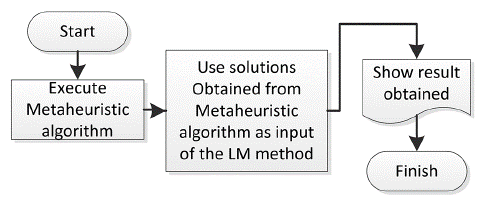Source: Authors

Figure 1 Flow chart of the hybrid methods used in this work.

Mathematical model of the system

For demonstrative purposes, an isotropic and homogeneous solid sphere of radius (a), with constant density (p) and specific heat (c) is considered. The heat conduction equation expressed in spherical polar coordinates assumes no variation for the (θ, φ ) coordinates. The rate of internal heat generation per unit volume at r = 0 is q(r") . The mathematical model is, thus, given by 1, where k is the thermal diffusivity of the substance; K is the thermal conductivity of the substance, and T (r,t)is the temperature. The relationship between k and K is given by k = K/p. Temperature at its boundary and in the initial condition is assumed to be 25°C.

Statement of the direct problem

It is possible to calculate the temperature distribution within a sphere homogeneously irradiated by microwaves. Here, we assume that all parameters of the above mathematical model are known, including the initial and boundary conditions. In the same way, we select three functional forms for the heat generation parameter, i.e. linear, sinusoidal and exponential.

Statement of the inverse problem

Based on the solution of the direct problem, it is possible to estimate properties such as heat capacity as well as internal volumetric heat generation parameter q' Q " within the solid sphere. Solving this problem requires experimental temperature readings at various radial positions within the sphere, and knowledge of the nature of the internal heat generation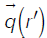. Nonetheless, they can be gathered using one or several temperature sensors.

Direct problem solution

This problem already has a well-known general analytical solution given in (Carslaw & Jaeger 1959) that yields the temperature profile within the sphere as shown in Equation (2), where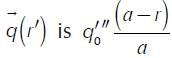for the linear form,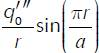for the sinusoidal form, and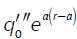for the exponential form. The term q0 is the magnitude of the internal volumetric heat generation term. In order to plot this solution, Equation (2) was normalized for the variables (T, r and, t) and the new ones are denoted by (T u , r u and, t u ) as shown in Equation (3), where each term is detailed in Equation (4).

Figure 2 shows the normalized theoretical temperature within the sphere as a function of the normalized radius and normalized time, as well as the profile temperature after a long time of irradiation. As expected, the temperature field is axisymmetric, having the maximum value at the center. For the figure, numbers on the curves represent the parameter t u . For the sake of brevity, the other two graphs for the sinusoidal and exponential case were omitted.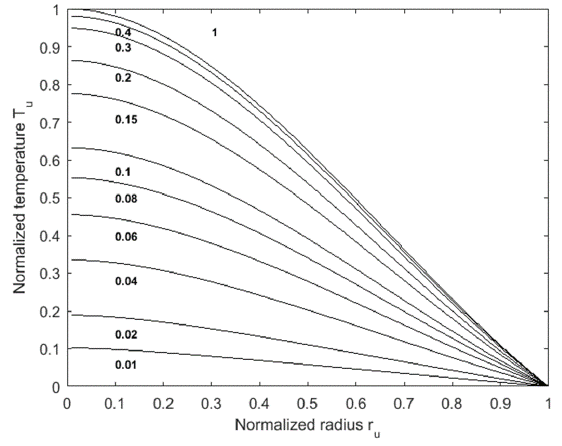Source: Authors

Figure 2 Normalized temperature in function of the normalized radius and normalized time for a sphere with linear internal heat generation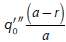. Numbers on the curves represent the normalized time (tu).

Simulating temperature measurements

We used synthetic temperature values for simulating real measured values, with the objective of checking the validity of this approach for a microwave heating process analysis. As expected, real temperature measurements may contain random errors. Here, such errors are assumed to be additive, uncorrelated, and normally distributed with zero mean and a constant standard deviation. Hence, we accept as valid the basic standard statistical assumptions proposed by Beck (Vere Beck & Kenneth 1977). Consequently, a white Gaussian noise (AWGN) was added to the theoretical normalized temperature to construct synthetic ones. In this case, SNR was kept constant at 30 dB. Figure 3 shows an example of the theoretical and measured temperatures for a given position and time. In the figure, temperature measurements at normalized time tu = 0,1, are shown as an example. For conciseness, the other two graphs for the sinusoidal and exponential case were omitted.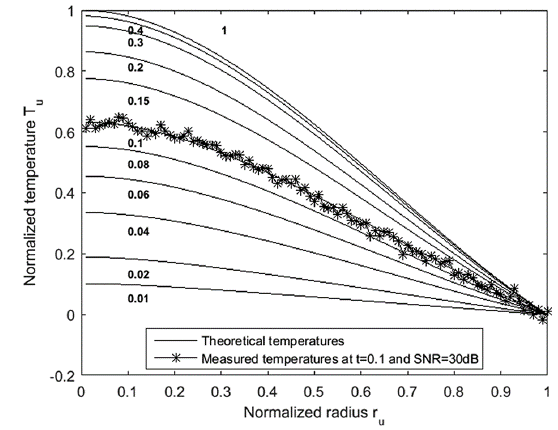Source: Authors

Figure 3 An example of simulated temperature measurements at tu = 0,1 with a SNR = 30 dB when the internal heat generation is linear. Numbers on the curves represent the normalized time (tu).

Objective function (OF)

The OF that provides the minimum variance in an inverse problem is the ordinary least squares (OLS) norm. In this work, we are going to take into consideration the case when the transient readings (Y) were taken from multiple sensors, as shown in Equation (5), where Y and T are vectors containing the synthetic (measured) and theoretical temperatures, respectively. It is worth remarking that due to the simulated nature of this work, whenever temperatures are labeled as "measured" they actually indicate simulated measurements generated by our model.

Error and RMS error

We considered throughout this work two metrics for the error. The reasoning behind this decision was that sometimes algorithms may converge to an estimated solution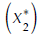distant to the theoretical one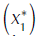, but for which the evaluation of the objective function (OF) is similar. Moreover, by using both metrics we can explain why in some cases the theoretical and estimated parameters exhibit a high percentage of error in the parameters, while having a low RMS error in the evaluation of the OF. Therefore, the first one was the error of the parameters themselves, as shown in equation 6, where theoretical parameters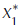are contrasted against the estimated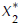ones (i.e.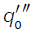״ and cp). The second one was the RMS error of the temperature profile, as shown in equation 7, where the theoretical temperature (T) is contrasted against the estimated temperature (Test). The latter was calculated with the parameters obtained through the optimization algorithms. In other words, the RMS error relates, in a way, the evaluation of the OF1 to OF2.

Results and analysis

This section is divided into two main subsections. The first one describes the application discussed above. Within this, the results obtained with the algorithms executed on the data (i.e. LM method, metaheuristic algorithms, and the hybrid algorithms) are presented. The second one is a performance analysis that was carried out with the results derived from all the algorithms.

A demonstrative example

Consider the microwave treatment of an isotropic and homogeneous silicon carbide (SiC) sphere with constant density, thermal conductivity, and specific heat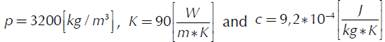respectively (Grup d'Innovació per la Millora de la Docência en Estructura Propietats i Processat de Materials n.d.). Its diameter is 2 [cm] and internal heat generation rate at r = 0 is 8000 [W/m3]. The temperature at its boundary is fixed at 25 °C. Furthermore, it is assumed that four sensors are located at unitary radius r u = 0,3, r u = 0,5, r u = 0,7 and r u = 1. The goal is to estimate c and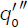, by solving the inverse problem. It is important to keep in mind that most of the reported data are normalized. Therefore, parameters c andobtained through the algorithm are also normalized. However, data shown within this section relate to their unnormalized equivalents.

LM method

Table 1 summarizes the results obtained with this algorithm. However, it is important to note that this algorithm converges under certain initial conditions (IC). If these IC are very far from the true values (i.e. 0.9 times the true value for the lower limit and 35 times for the higher limit in the linear case), LM does not converge. Table 2 shows the ranges tested for this particular problem.

Table 1 Estimated parameter for the three sources, their error and the RMS error obtained with the LM method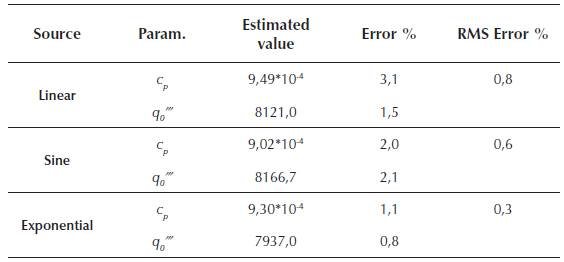Source: Authors

Table 2 Range of initial conditions in which the LM algorithm converges, where x* is the real value of the parameter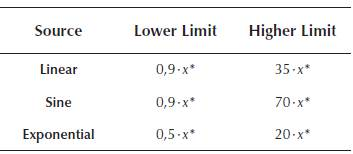Source: Authors

Metaheuristic algotithms

This time, SOA, VS and WAM were used to find parameters c p and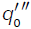. To do so, several tests were carried out. The range of the IC where the metaheuristic algorithms were tested is shown in Table 3. The parameters used in each algorithm are shown in Table 4. Each algorithm was repeated 50 times and resulting data are summarized in Table 5.

Table 3 Range of initial conditions in which the metaheuristics were tested, where x* is the real value of the parameter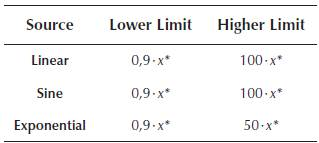Source: Authors

Table 4 Parameters used in the metaheuristic algorithms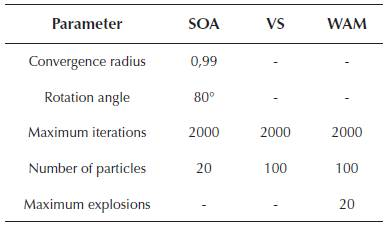Source: Authors

Table 5 Estimated parameters for the three sources, with some basic statistics of the result.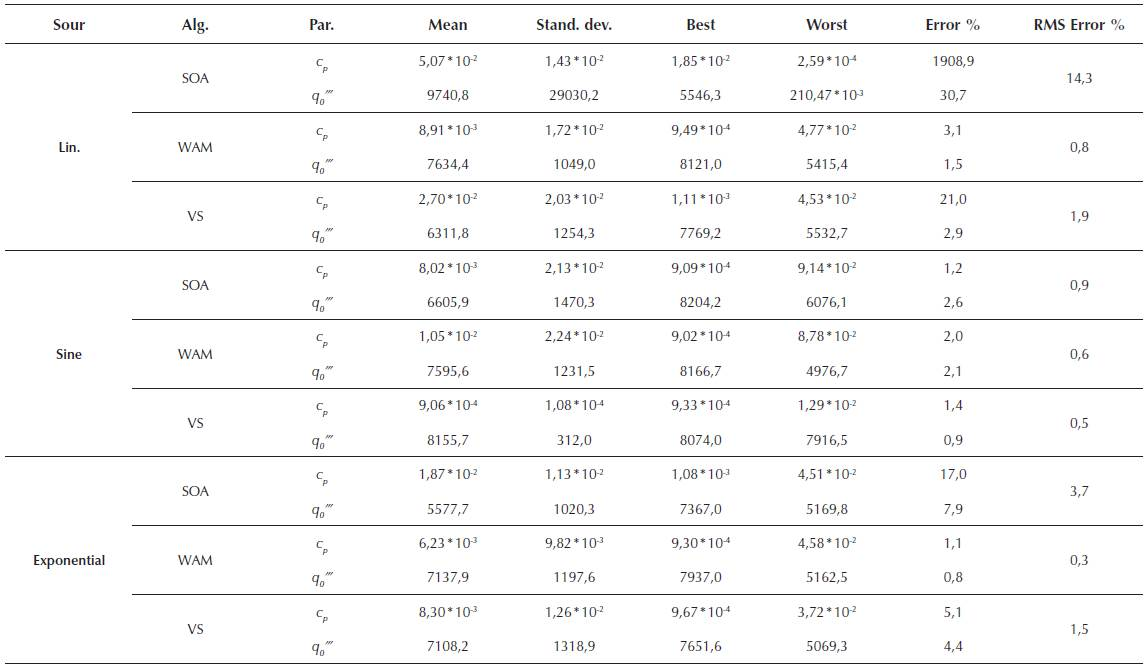Source: Authors

Hybrid algorithms

In this scenario, the hybrid algorithms were used, considering the same parameters in Table 3 and Table 4. Tests were repeated 50 times and results were summarized in Table 6. As data show, using heuristics to identify proper initial points for LM leads to improved results (Table 2 and Table 5). Thus, these hybrids are more robust than the single use of heuristics.

Table 6 Estimated parameters for the three sources, with some basic statistics of the result.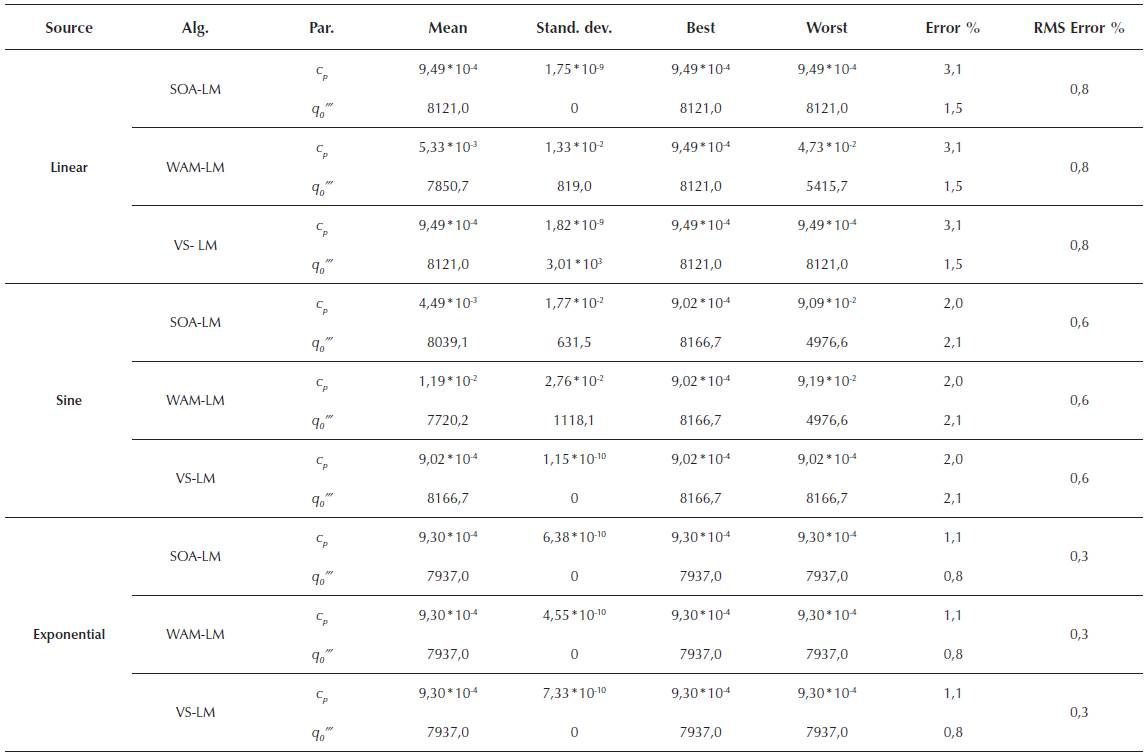Source: Authors

Algorithms performance

A performance comparison of all algorithms is carried out. Results are shown in Table 7, Table 8 and Table 9. In these tables, it can be seen that LM algorithm requires less time and iterations than the metaheuristic and hybrid algorithms. Besides, hybrid algorithms require, in average, 10 seconds and 19 iterations more than metaheuristics. Additionally, Table 10 and Table 11 show an analysis of the accuracy based on the results of all experiments (i.e. 900). It is important to keep in mind that a guess is considered right when the parameter estimation resides within a 10% confidence interval. If it is outside, the guess is deemed wrong. Through these tables, we show that the results obtained with the hybrid algorithms improve the results obtained with the metaheuristics in over 60%.

Table 7 Number of iterations and computation time in LM method for all sources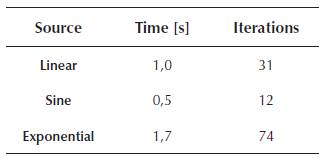Source: Authors

Table 8 Mean and standard deviation (Std) of the number of iterations and computation time for all metaheuristics and sources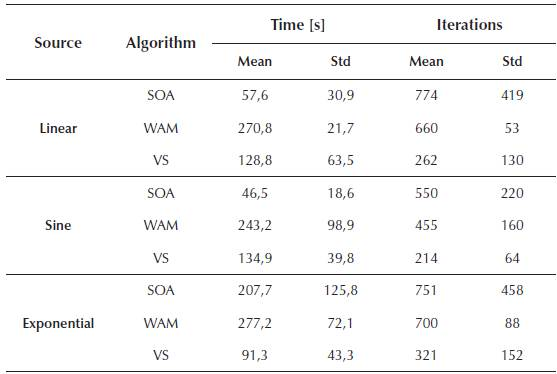Source: Authors

Table 9 Mean and standard deviation (Std) of the number of iterations and computation time for all hybrids and sources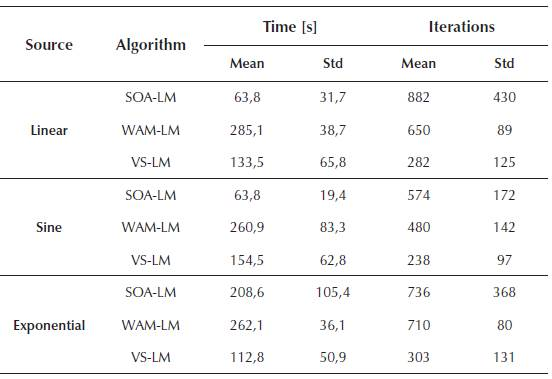Source: Authors

Table 10 An analysis of the prediction accuracy for the heuristic algorithms. A guess is considered as right when it resides within a 10% confidence interval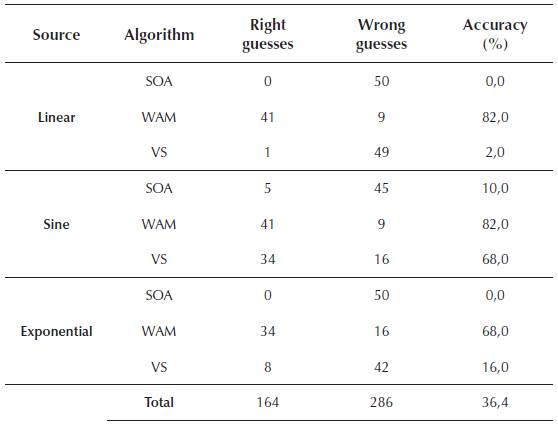Source: Authors

Table 11 An analysis of the prediction accuracy for the hybrid algorithms. A guess is considered as right when it resides within a 10% confidence interval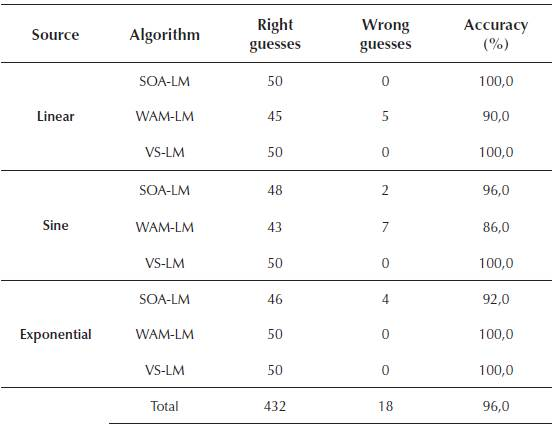Source: Authors

Conclusions

This article described the estimation of the internal volumetric heat generation and the heat calorific capacity parameters during microwave heating of a solid spherical sample. We used three functional forms (linear, sinusoidal, and exponential) for the electromagnetic field. We incorporated White Gaussian noise into the direct problem to simulate a more realistic situation. Then, we solved the inverse problem through three different kinds of approaches. The first one used a traditional deterministic Levenberg-Marquardt (LM) algorithm. The second one used modern stochastic metaheuristics (SOA-VS-WAM). The final one was the proposed hybrid of the first two. Results show that LM converges to the expected solutions only if the initial conditions (IC) comply with specific ranges. However, LM only required 0,6% of the time employed by metaheuristics and hybrids. Nonetheless, through metaheuristics we can expand the range of the IC by 42 times. However, these methods only yield an accuracy of about 36%. Thus, by using hybrid algorithms we can merge the best of both approaches, increasing the accuracy of metaheuristics by about 60%, while only requiring about 6% more time. Therefore, we highly recommend to use this kind of hybrids for these inverse problems.

References

Bermeo, L. et al ., 2015. Estimation of the particle size distribution of colloids from multiangle dynamic light scattering measurements with particle swarm optimization. Ingeniería e Investigación, 35(1), pp.49-54. [ Links ]

Bermeo Varon, L., Barreto Orlande, H. & Eliçabe, G., 2015. Estimation of state variables in the hyperthermia therapy of cancer with heating imposed by radiofrequency electromagnetic waves. International Journal of Thermal Sciences, 98, pp.228-236. [ Links ]

Carslaw, H.S. & Jaeger, J.C., 1959. The flow heat in a sphere and cone. In Conduction of heat in solids. London: Oxford University Press, pp. 230-244. [ Links ]

Cebo-Rudnicka, A., Malinowski, Z. & Buczek, A., 2016. The influence of selected parameters of spray cooling and thermal conductivity on heat transfer coefficient. International Journal of Thermal Sciences, 10, pp.52-64. [ Links ]

Chen, B. et al ., 2016. Identification of the thermal conductivity coefficients of 3D anisotropic media by the singular boundary method. International Journal of Heat and Mass Transfer, 100, pp.24-33. [ Links ]

Cui, M. et al ., 2016. A modified Levenberg-Marquardt algorithm for simultaneous estimation of multi-parameters of boundary heat flux by solving transient nonlinear inverse heat conduction problems. International Journal of Heat and Mass Transfer, 97, pp.908-916. Available at: http://linkinghub.elsevier.com/retrieve/pii/S0017931015317932. [ Links ]

Dogan, B. & Olmez, T., 2015. A new metaheuristic for numerical function optimization: Vortex Search algorithm. Information Sciences, 293, pp.125-145. [ Links ]

Friedl, G. & Kuczmann, M., 2015. A New Metaheuristic Optimization Algorithm, the Weighted Attraction Method. Acta Technica Jaurinensis, 8(3), pp.257-266. Available at: http://acta.sze.hu/index.php/acta/article/view/381. [ Links ]

Giraldo, E., Peluffo, D. & Castellanos, G., 2012. Weighted time series analysis for electroencephalographic source location. DYNA, 79(176), pp.64-70. [ Links ]

Grup d'Innovació per la Millora de la Docência en Estructura Propietats i Processat de Materials, Materials - Silicom Carbide. Available at: http://www.ub.edu/cmematerials/es/content/carburo-de-silicio-sic [Accessed June 16, 2016]. [ Links ]

Hào, D. et al ., 2017. Determination of a term in the right-hand side of parabolic equations. Journal of ComFutational and Applied Mathematics, 309, pp.28-43. [ Links ]

Hussein, M.S. & Lesnic, D., 2014. Determination of the time-dependent thermal conductivity in the heat equation with spacewise dependent heat capacity. In 6th International Conference on Finite Difference Methods, FDM. pp. 217- 224. [ Links ]

Kvasov, D.E. & Mukhametzhanov, M.S., 2017. Metaheuristic vs. deterministic global optimization algorithms: The univariate case. Applied Mathematics and ComFutation. [ Links ]

Kvasov, D.E. & Sergeyev, Y.D., 2014. Deterministic approaches for solving practical black-box global optimization problems. Advances in Engineering Software. [ Links ]

Mohebbi, F. & Sellier, M., 2016. Estimation of thermal conductivity, heat transfer coefficient, and heat flux using a three dimensional inverse analysis. International Journal of Thermal Sciences, 99, pp.258-270. [ Links ]

Mohebbi, F. & Sellier, M., 2016. Parameter estimation in heat conduction using a two-dimensional inverse analysis. In International Journal of ComFutational Methods in Engineering Science and Mechanics. pp. 1-14. [ Links ]

Necati Ozisik & Orlande, H., 2000. Techniques for solving inverse heat transfer problems. In Inverse Heat Transfer -Fundamentals and applications. New York: Taylor & Francis, pp. 35-58. [ Links ]

Nedin, R., Nesterov, S. & Vatulyan, A., 2016. Identification of thermal conductivity coefficient and volumetric heat capacity of functionally graded materials. International Journal of Heat and Mass Transfer, 102, pp.213-218. [ Links ]

Sergeyev, Y.D. & Kvasov, D.E., 2017. Deterministic Global Optimization: An Introduction to the Diagonal Approach, Springer-Verlag New York. [ Links ]

Strongin, R.G. & Sergeyev, Y.D., 2000. Global Optimization with Non-Convex Constraints: Sequential and Parallel Algorithms 1st ed., Springer US. [ Links ]

Tamura, K. & Yasuda, K., 2011. Spiral Multipoint Search for Global Optimization. In 207 7 70th International Conference on Machine Learning and AFFlications and WorkshoFs. Anchorage, AK: IEEE, pp. 470-475. Available at: http://ieeexplore.ieee.org/lpdocs/epic03/wrapper.htm?arnumber=6147022. [ Links ]

Tutcuoglu, A., Majidi, C. & Shan, W., 2016. Nonlinear thermal parameter estimation for embedded internal Joule heaters. International Journal of Heat and Mass Transfer, 97, pp.412-421. [ Links ]

Vere Beck, J. & Kenneth, A., 1977. Introduction To Linear Estimation. In Parameter Estimation in Engineering and Science. New York: John Wiley & Sons, pp. 130-212. [ Links ]

Wang, B. & Liu, J., 2016. Recovery of thermal conductivity in two-dimensional media with nonlinear source by optimizations. Applied Mathematics Letters, 60, pp.73-80. [ Links ]

Wang, L. et al ., 2017. Identification of the boundary heat transfer coefficient from interior measurement of temperature field. Applied Mathematics Letters, 63, pp.6-13. [ Links ]

Yang, Y., Chen, W. & Lee, H., 2011. A nonlinear inverse problem in estimating the heat generation in rotary friction welding. Numerical Heat Transfer; Part A: Applications, 59(2), pp.130-149. [ Links ]

Zhigljavsky, A. & Žilinskas, A., 2008. Stochastic Global Optimization, Springer US. [ Links ]

How to cite: García, E., Amaya, I., Correa, R. (2018). Internal volumetric heat generation and heat capacity prediction during a material electromagnetic treatment process using hybrid algorithms. Ingeniería e Investigación, 38(1),74-82. DOI: 10.15446/ing.investig.v38n1.64225

Received: April 21, 2017; Accepted: October 24, 2017This is an open-access article distributed under the terms of the Creative Commons Attribution License